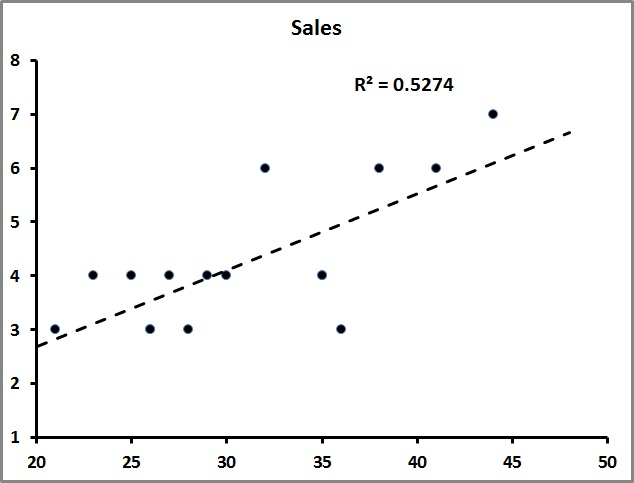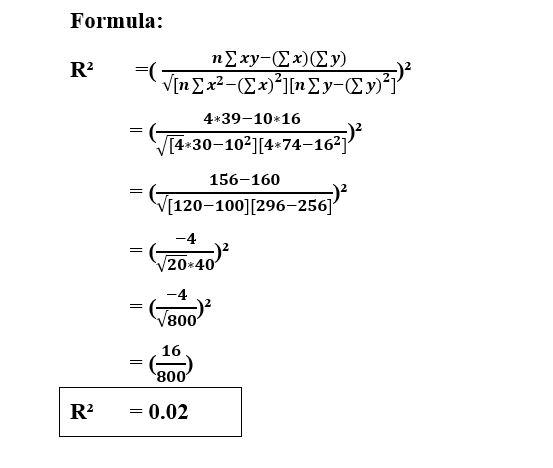### Co-efficient of Determination

• date 11th July, 2019 |
• by Prwatech |

# Co-efficient of Determination Tutorial

Co-efficient of Determination tutorial, Are you the one who is looking for the best platform which provides information about the Co-efficient of Determination in data science? then welcome to the land of Co-efficient of Determination tutorial by Prwatech. Before proceeding, we recommend you to go through our previous blog in this series on the need for a margin of error tutorial in data science.

Are you the one who is looking for the best platform to learn Data science tutorials? Or Are you the one who is dreaming to become an expert data scientist? Then stop dreaming yourself, start taking Data Science training from Prwatech, who can help you to guide and offer excellent training with highly skilled expert trainers with the 100% placement. Follow the below-mentioned Co-efficient of Determination tutorial in data science and enhance your skills to become pro Data Scientist.

## Coefficient of Determination Definition

The coefficient of determination is a unit used in statistical analysis that assesses how well a model explains and predicts future outcomes. It indicates the level of explained variability in the data set. The coefficient of determination, also known as “R-squared,” is used as a guideline to calculate the accuracy of the model.

One way to interpret this figure is to say that the variables included in a given model explain approximately x% of the observed variation.

So, if the R2 = 0.50, then approximately half of the observed variation can be explained by the model.### Why we need a Coefficient of Determination?

The coefficient of determination is used to explain how much variability of 1 factor can cause by its relationship to another factor. It is relied on highly on trend analysis and is denoted as a value between 0 and 1.

The closer the value towards 1, the better the fit will be, or the relationship, between the two factors.

The coefficient of determination is the square of the correlation coefficient (R) that allows it to display the degree of linear correlation between the 2 variables.

This correlation is also known as the “goodness of fit.”A value of 1.0 displays a perfect fit, and it is thus a reliable model for future forecasts, indicating that the model explains all of the variations observed. A value of 0, on the other hand, will denote that, the model fails to accurately model the data at all. In economics, an R2 value above 0.60 is seen as worthwhile.

## Co-efficient of Determination Example## Co-efficient of Determination FormulaInterested in securing your career as a Data Scientist?

Be a part of our Co-efficient of Determination advanced tutorials-based career programs of Data Science training institute in Bangalore by qualified specialists.

### Quick Support